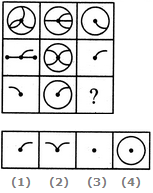# Non Verbal Reasoning - Figure Matrix - Discussion

### Discussion :: Figure Matrix - Section 1 (Q.No.5)

In each of the following questions, find out which of the answer figures (1), (2), (3) and (4) completes the figure matrix ?

5.

Select a suitable figure from the four alternatives that would complete the figure matrix.[A]. 1 [B]. 2 [C]. 3 [D]. 4

Explanation:

The third figure in each row comprises of the parts common to the first two figures.

 Makku said: (Nov 24, 2011) Explain the problems this type how to solve it clearly.

 Please Enter Your Name. said: (Mar 28, 2013) OK for the first figures there is something and then for the second figure there is another shape that has everything from the first shape except one thing and for the third shape you have to find that one thing that is missing;). Hope that helped all.

 Thiago said: (Dec 7, 2014) For that logic, answer 2 would also be correct, because it also comprises of the parts common to the first two figures.

 Name said: (Mar 3, 2015) In each row, the first two figures don't have any pattern or relationship. But the third figure only includes the parts where the first two shapes 'overlap'.

 Mukesh said: (Nov 30, 2015) Find what is common in both figures is answer as third figure leave all that is not both figures.

 Tom said: (May 24, 2016) Good point. Only the overlap figure stays back. Thanks.

 Saranya said: (Aug 13, 2016) Please explain how to solve this type of problems.

 The Man Of Steel said: (Sep 11, 2016) The answer is correct. There is nothing common in the 3rd figure matrix.

 Rachu said: (Sep 25, 2018) 2 is the correct answer.

 Arunava said: (Oct 22, 2018) Good point. Only overlap figure stays back.

 Vidhya said: (Jun 19, 2019) Why can't it be 2?

 Prakhar said: (Nov 10, 2019) Please give some trick for it.

 Prashanth said: (Feb 18, 2020) @Vidhya 2 cannot become the answer as it's consists of both a mixture of 1 & 2 and not the common thing from them. Try to find which is common to both 1&2. You will get the correct answer as an option C. Hope you can get it I think.

 Prithi said: (Jun 12, 2020) Yes, 2 is the correct answer.

 Sirisha said: (Aug 26, 2020) In the first two figures, we have to see common curves So, 3 is correct.

 Shaina said: (Nov 15, 2020) I am not getting this, Could someone please explain this to me?

 Jack said: (Mar 4, 2021) Why not 1? Explain